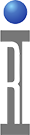Many applications require low current measurements below the 1 uA specified accuracy of the DB line low current measurement. In fact, The DB line's current measurement performance can be improved with calibration to almost 1000 times better accuracy. Less than 10 nA performance is achievable with a simple calibration procedure.

Typical DC tester resources follow a "Slope/Offset" error performance. When measuring a calibration artifact, the difference between the measured value and the real value can be modeled by a y=mx+b. In this case specifically: current error = m(stimulus voltage) + (current offset error). We will go through an example and see how the error can be measured and then used to correct any measured value to approximately 1 nA accuracy.

Here is a test plan panel used to calibrate DB1 using 4.87M Ohm resistor. Note how it ramps voltage and compares the actual measured current to the ideal or perfect current. The measured current is DB1 current. The ideal current is the instrument state source divided by the resistor value. The error is saved as DB1 Current Offset.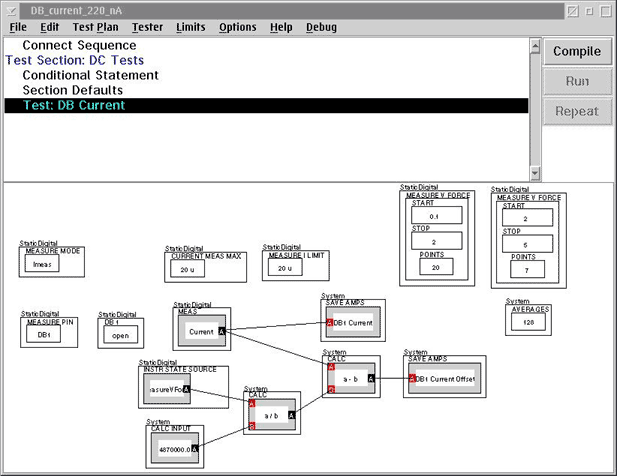Here is the measured DB1 current offset. Note the value is a straight line. That is what enables us to use the slope/offset method. The Y axis (current) intercept is -100.8 nA and the (slope Delta current/delta voltage) is 7nA/5V. The measured plot was actually done at a voltage sweep from -2 to +5 volts. This makes it easy to identify the intercept (current when V=0).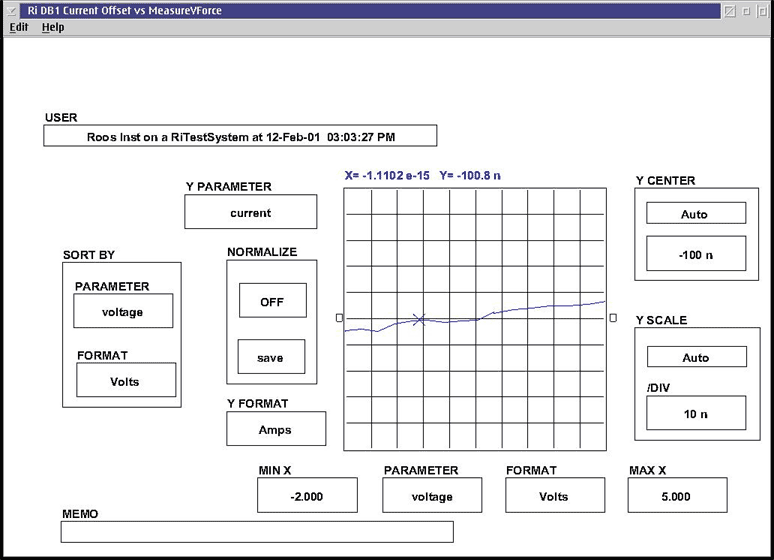From this we have an error equation of:

error = 1.4e-9(V force) + (-108e-9)

Therefore to get the correct value we use this equation:

calibrated value = measured value - (1.4e-9(V force) + (-108e-9)).

In addition this error is consistent across each bank of DB lines, since each bank share the same tester resource. DB 1-9 share the same error, and DB 9-16 share the same error. A real time calibration can be implemented using a precision resistor on a DUT board. This panel shows an example. Note that the slope is established by subtracting the error current at 5V force from the error current at 0V force. The offset is just the error current at 0V force.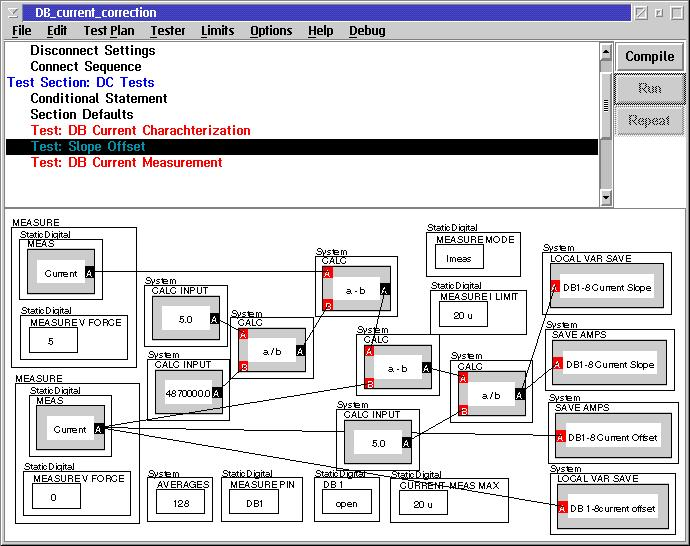Below is a test panel that shows a measurement and a correction using the Local Variables from the preceding panel.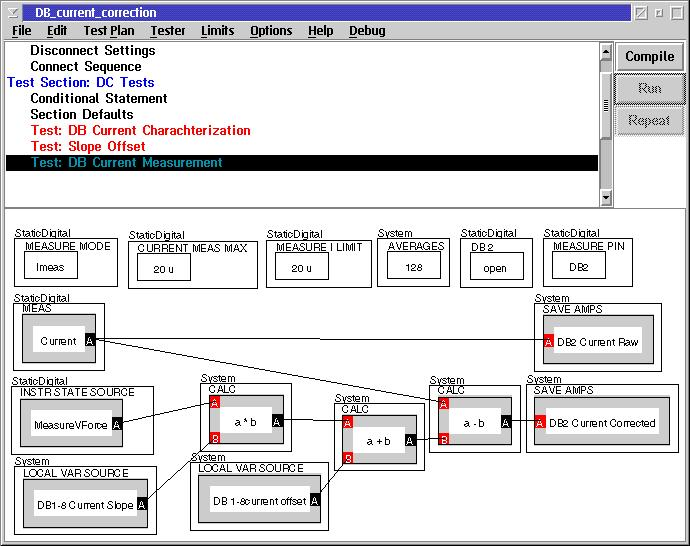A user has the option of implementing the offset or the offset and slope error correction. It is possible, (and only requires one cal measurement) to ignore the slope and only use the offset. Implementation of offset only would allow approximately +/- 10 nA accuracy and slope offset would allow +/- 3 nA accuracy. Attached is a test plan that includes the panel to prove DB linearity, to establish slope and offset, and to make the corrected measurement.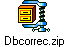https://roos.com/docs/MFEY-4TVPMC
ROOS INSTRUMENTS CONFIDENTIAL AND PROPRIETARY